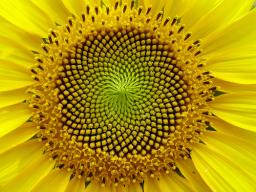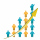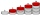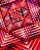# Sequence

Between numbers 1 and 53 insert n members of the arithmetic sequence that its sum is 702.

n =  26

### Step-by-step explanation:Did you find an error or inaccuracy? Feel free to write us. Thank you!## Related math problems and questions:

• AS sequenceIn an arithmetic sequence is given the difference d = -3 and a71 = 455. a) Determine the value of a62 b) Determine the sum of 71 members.
• Two geometric progressionsInsert several numbers between numbers 6 and 384 so that they form with the given GP numbers and that the following applies: a) the sum of all numbers is 510 And for another GP to apply: b) the sum of entered numbers is -132 (These are two different geome
• Insert 3Insert five arithmetic progression members between -7 and 3/2.
• Finite arithmetic sequenceHow many numbers should be inserted between the numbers 1 and 25 so that all numbers create a finite arithmetic sequence and that the sum of all members of this group is 117?
• InsertInsert five numbers between 8 and 27 such numbers that, with two given ones, they form the first seven members of the geometric sequence.
• The sumThe sum of the first 10 members of the arithmetic sequence is 120. What will be the sum if the difference is reduced by 2?
• Insert into GPBetween numbers 5 and 640, insert as many numbers to form geometric progression so the sum of the numbers you entered will be 630. How many numbers must you insert?
• Three members GPThe sum of three numbers in GP (geometric progression) is 21 and the sum of their squares is 189. Find the numbers.
• Geometric sequence 4It is given geometric sequence a3 = 7 and a12 = 3. Calculate s23 (= sum of the first 23 members of the sequence).
• GP membersThe geometric sequence has 10 members. The last two members are 2 and -1. Which member is -1/16?
• A bookA book contains 524 pages. If it is known that a person will select any one page between the pages numbered 125 and 384, find the probability of choosing the page numbered 252 or 253.
• Sum of membersWhat is the sum of the first two members of the aritmetic progression if d = -4.3 and a3 = 7.5?
• Sequence 3Write the first 5 members of an arithmetic sequence: a4=-35, a11=-105.
• AP - simpleFind the first ten members of the sequence if a11 = 132, d = 3.
• Five elementThe geometric sequence is given by quotient q = 1/2 and the sum of the first six members S6 = 63. Find the fifth element a5.
• SequenceIn the arithmetic sequence is a1=-1, d=4. Which member is equal to the number 203?
• Insert 4Insert three arithmetic means between 3 and 63.